# IFERROR (& IFNA) XLOOKUP

This tutorial will demonstrate how to handle XLOOKUP #N/A errors in Excel. If your version of Excel does not support XLOOKUP, read how to error handle VLOOKUPs instead.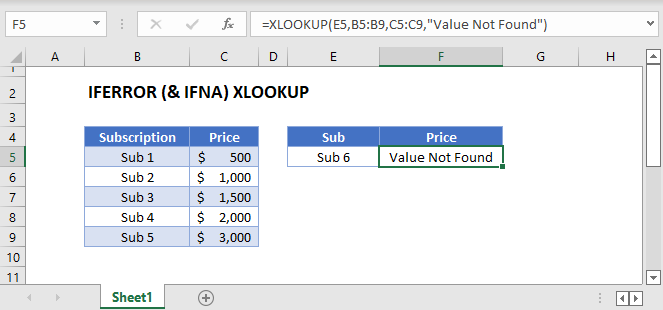## XLOOKUP: #N/A Error-Handling

Unlike VLOOKUP, XLOOKUP has the built-in option to handle #N/A Errors. By entering a value into the 4th argument, the XLOOKUP Function will replace a #N/A error with the specified value.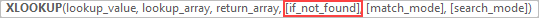In this example, we replace the #N/A Error with the text “Value Not Found,”.

``=XLOOKUP(E3,B3:B7,C3:C7,"Value Not Found")``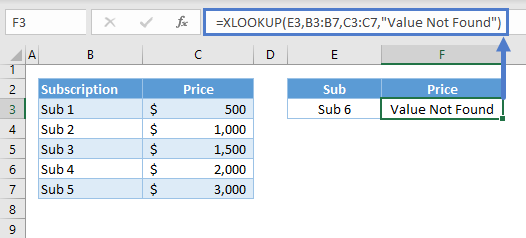Or we can also set it to 0:

``=XLOOKUP(E3,B3:B7,C3:C7,0)``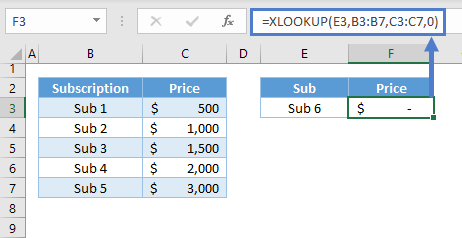Note: The XLOOKUP Function requires at least three arguments: lookup value, lookup array and return array. By default, the XLOOKUP Function finds an exact match from the top of the lookup array going down (i.e., top-down). Once it finds a match, it returns the corresponding value from the return array. Otherwise, it returns an error.

## Nested XLOOKUP: 2 Sheets at Once

Instead of a customized value, we can nest another XLOOKUP in the 4th argument of the XLOOKUP Function to perform a lookup among multiple sets of data that are stored in different sheets.

``=XLOOKUP(E3,B3:B7,C3:C7,XLOOKUP(E3,'2 Sheets - Data 2'!B3:B7,'2 Sheets - Data 2'!C3:C7))``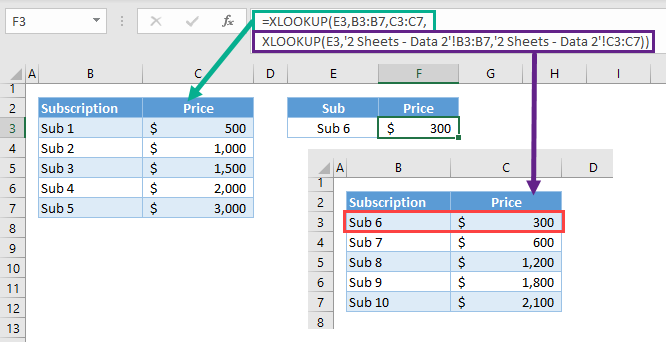## XLOOKUP & IFERROR

For arithmetic calculations involving XLOOKUP, we can use the IFERROR Function to handle all types of error.

``=IFERROR(1.5*XLOOKUP(E3,B3:B7,C3:C7),"Value Not Found")``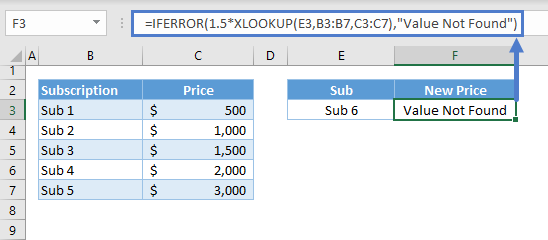The #N/A Error handling of the XLOOKUP Function won’t work on the above scenario:

``=1.5*XLOOKUP(F3,C3:C7,D3:D7,"Value Not Found")``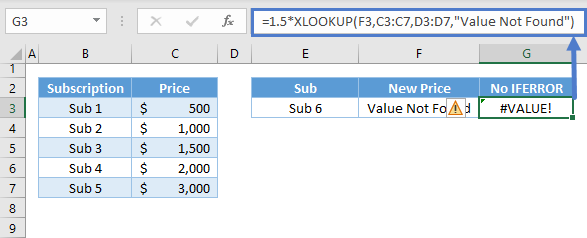### Excel Practice Worksheet

Practice Excel functions and formulas with our 100% free practice worksheets!